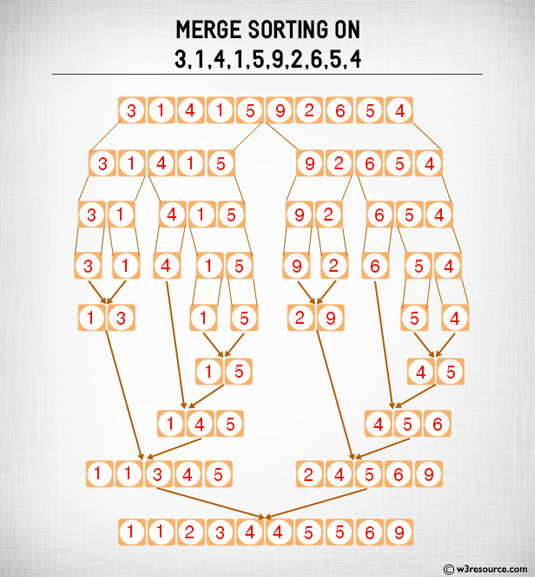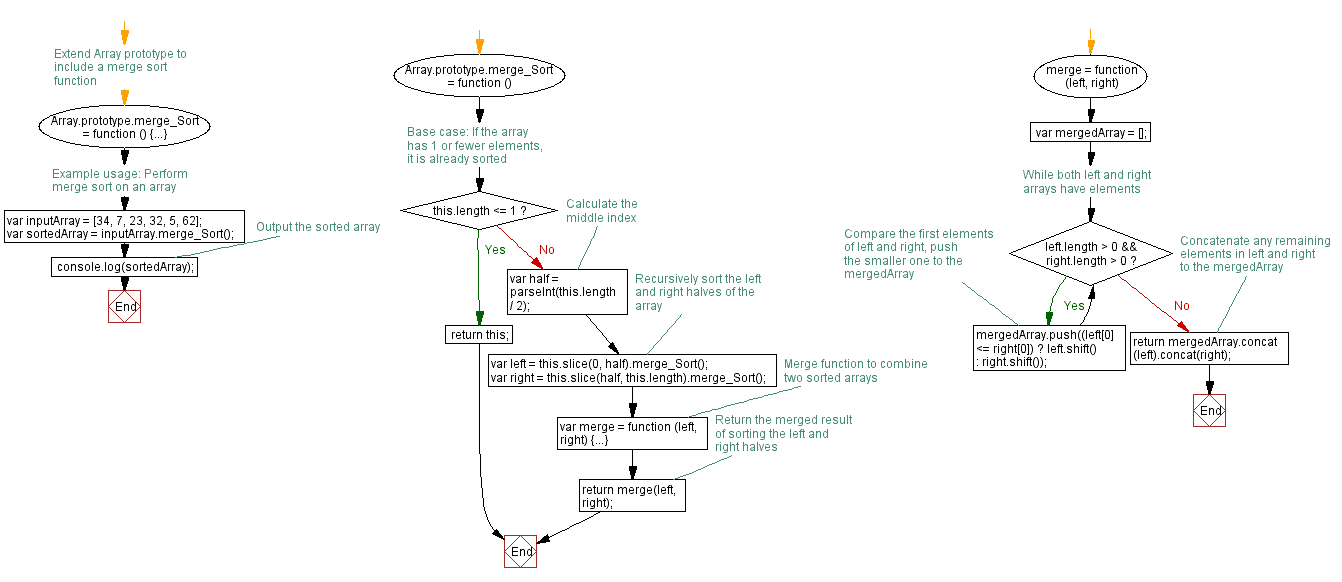# JavaScript: Marge sort - recursion

## JavaScript Function: Exercise-9 with Solution

Write a merge sort program in JavaScript.

Sample array : [34,7,23,32,5,62]
Sample output : [5, 7, 23, 32, 34, 62]

Pictorial Presentation:Sample Solution:-

HTML Code:

``````<!DOCTYPE html>
<html>
<meta charset="utf-8">
<title>Merge sort - recursion</title>
<body>

</body>
</html>
```
```

JavaScript Code:

``````Array.prototype.merge_Sort = function () {
if (this.length <= 1)
{
return this;
}

var half = parseInt(this.length / 2);
var left = this.slice(0, half).merge_Sort();
var right = this.slice(half,     this.length).merge_Sort();
var merge = function (left, right)
{
var arry = [];
while (left.length > 0 && right.length > 0)
{
arry.push((left <= right) ? left.shift() : right.shift());
}
return arry.concat(left).concat(right);
};

return merge(left, right);
};

var a = [34,7,23,32,5,62];
console.log(a.merge_Sort());
```
```

Output:

```[5,7,23,32,34,62]
```

Flowchart:Live Demo:

See the Pen javascript-recursion-function-exercise-9 by w3resource (@w3resource) on CodePen.

Improve this sample solution and post your code through Disqus

What is the difficulty level of this exercise?

Test your Programming skills with w3resource's quiz.

﻿

## JavaScript: Tips of the Day

Add padding to the beginning of a string

```const name = 'Minta Lofton';